# Blog Archives

## Ryacas version 1.0.0 released!

It is with great pleasure that I can announce that Ryacas version 1.0.0 is now released to CRAN (https://cran.r-project.org/package=Ryacas). I wish to thank all co-authors: Rob Goedman, Gabor Grothendieck, Søren Højsgaard, Grzegorz Mazur, Ayal Pinkus. It means that you can install the package by (possible after binaries have been built): install.packages("Ryacas") Followed by: library(Ryacas) (The source code is available at https://github.com/mikldk/ryacas/.) Now you have the yacas computer algebra...

## How much pizza and how much frozen yogurt? …with Gröbner bases

In a recent blog post I tried to get yacas to solve a system of polynomial equations. Unfortunately it could not do that, so I solved it numerically instead. Now it is possible – together with many other systems of polynomial equations thanks to fixing a small error in yacas. It has now been fixed, also in Ryacas (development version), so hurry up and...

## Approximating small probabilities using importance sampling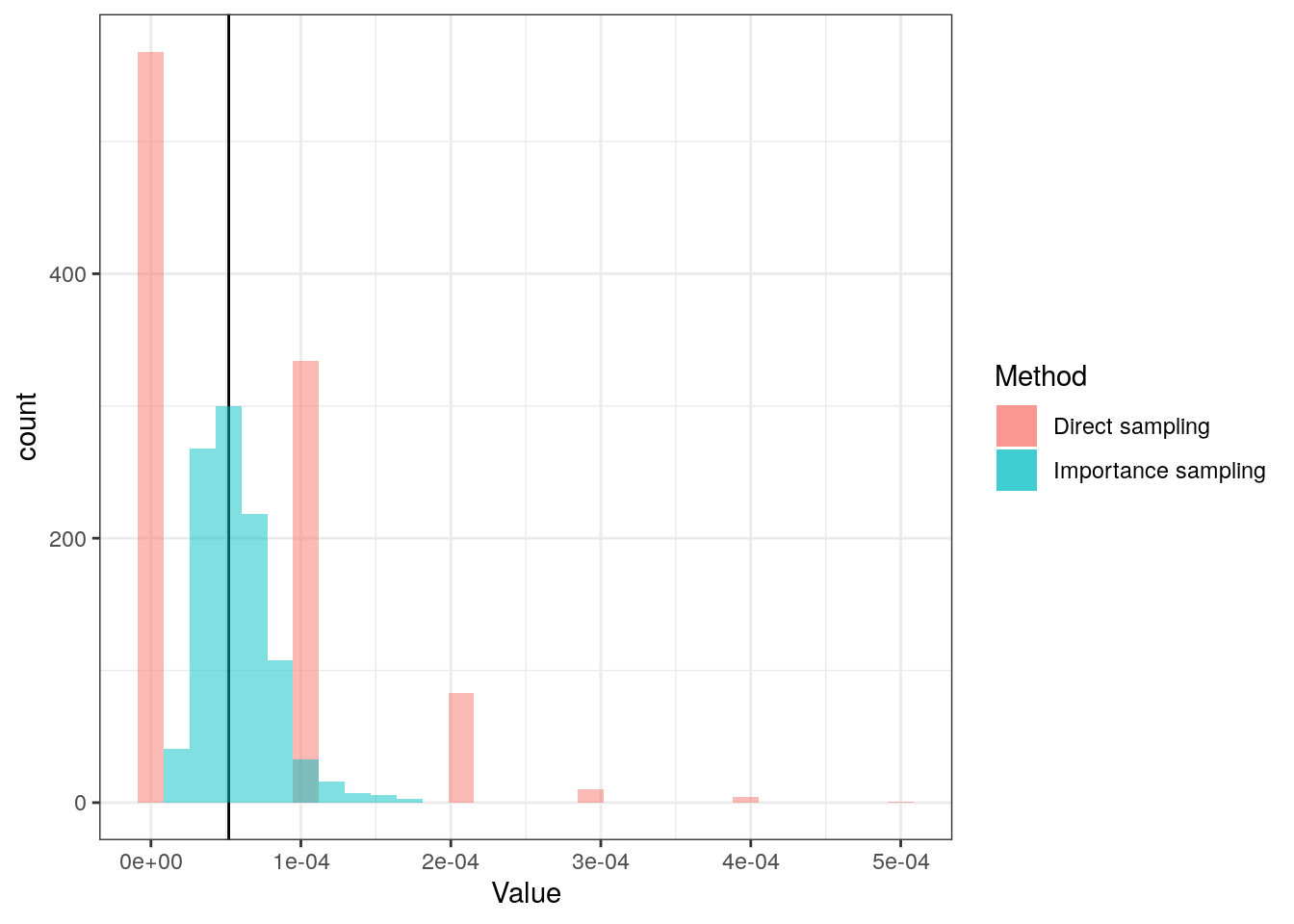Box plots are often used. They are not always the best visualisation (e.g. is bi-modality not visible), but I will not discuss that in this post. Here, I will use it as an example of Importance sampling that is a technique to estimate tail probabi...

## Prediction intervals for Generalized Additive Models (GAMs)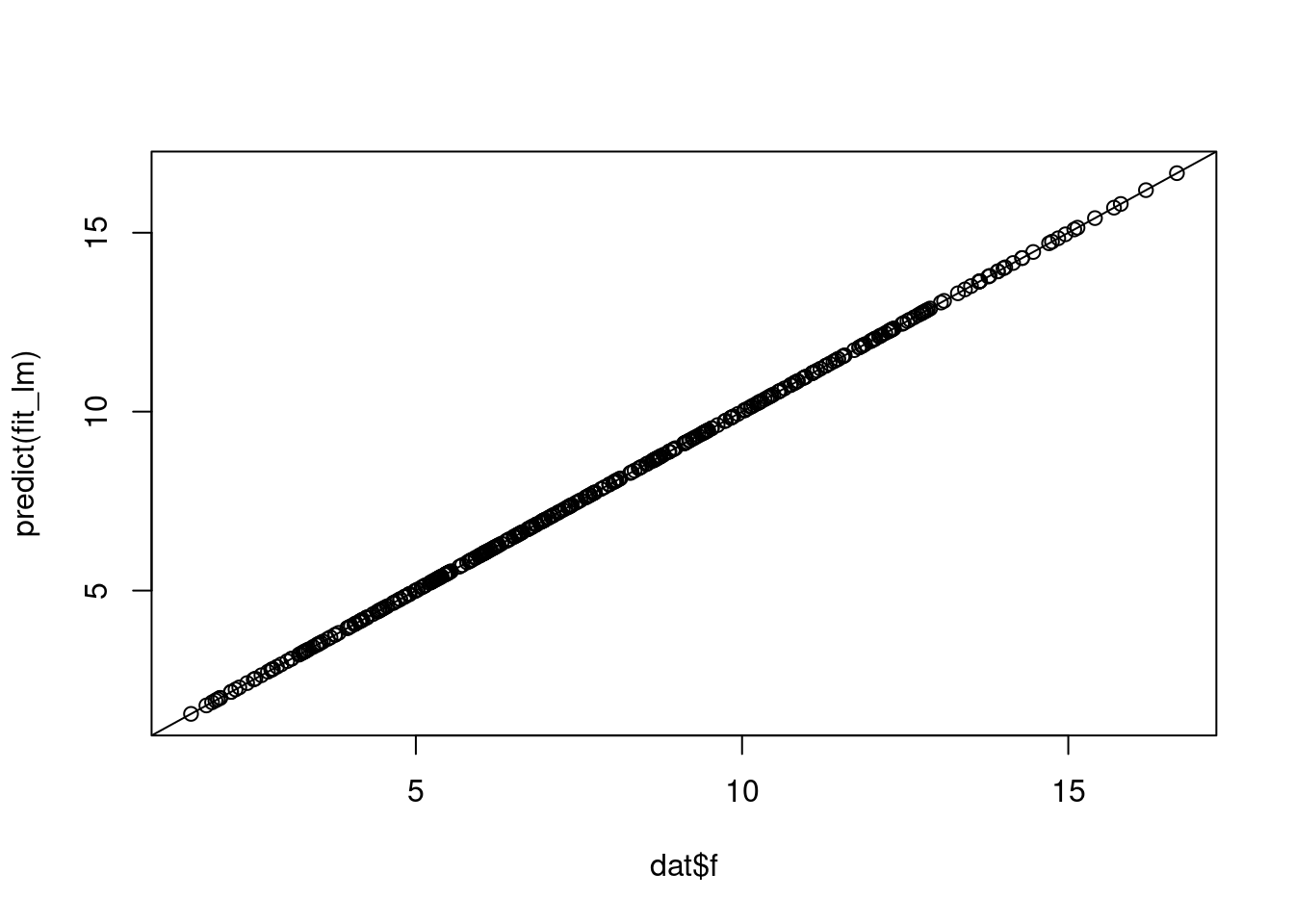Finding prediction intervals (for future observations) is something different than finding confidence intervals (for unknown population parameters). Here, I demonstrate one approach to doing so. First we load the library and simulate some data: library(mgcv) set.seed(1) dat

## Correlation is not transitive, in general at least: A simulation approach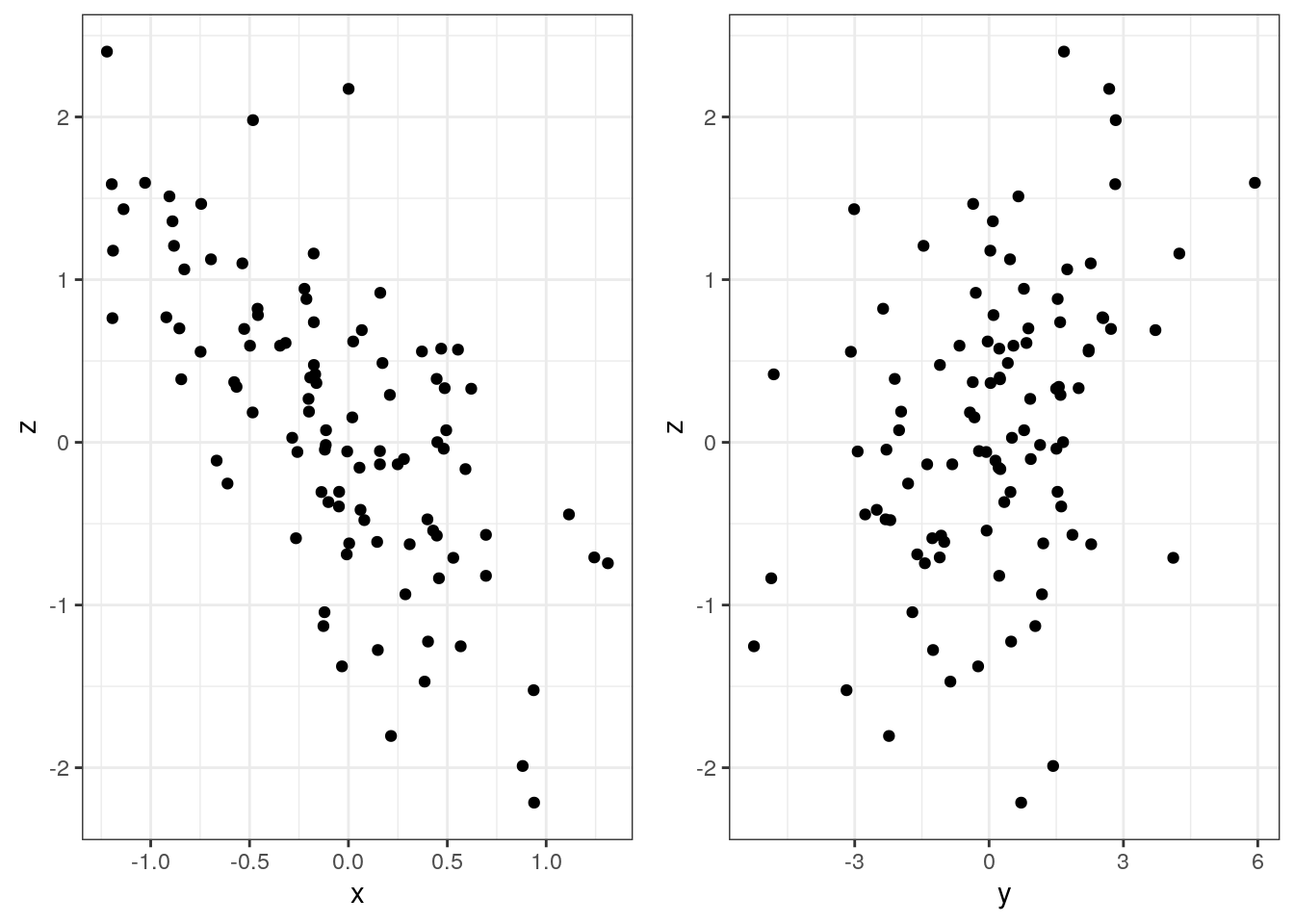Let $$\rho_{XY}$$ be the correlation between the stochastic variables $$X$$ and $$Y$$ and similarly for $$\rho_{XZ}$$ and $$\rho_{YZ}$$. If we know two of these, can we say anything about the third? In a recent blog post I dealt with the problem mathematically and I used the concept of a partial correlation coefficient. Here I will take a simulation approach. First z is simulated. Then...

## Automatic ‘testthat’ test skeletons with new R package ‘roxytest’ extending ‘roxygen2’

It is important to test software. One approach is unit-testing, and for R packages this can e.g. be done using testthat. It is also important to document software. For R packages roxygen2 is really helpful: It enables you to write documentation in the code file in the R/ folder where the function is implemented. And then roxygen2 takes care of handling the Rd files in...

## Correlation is not transitive, in general at least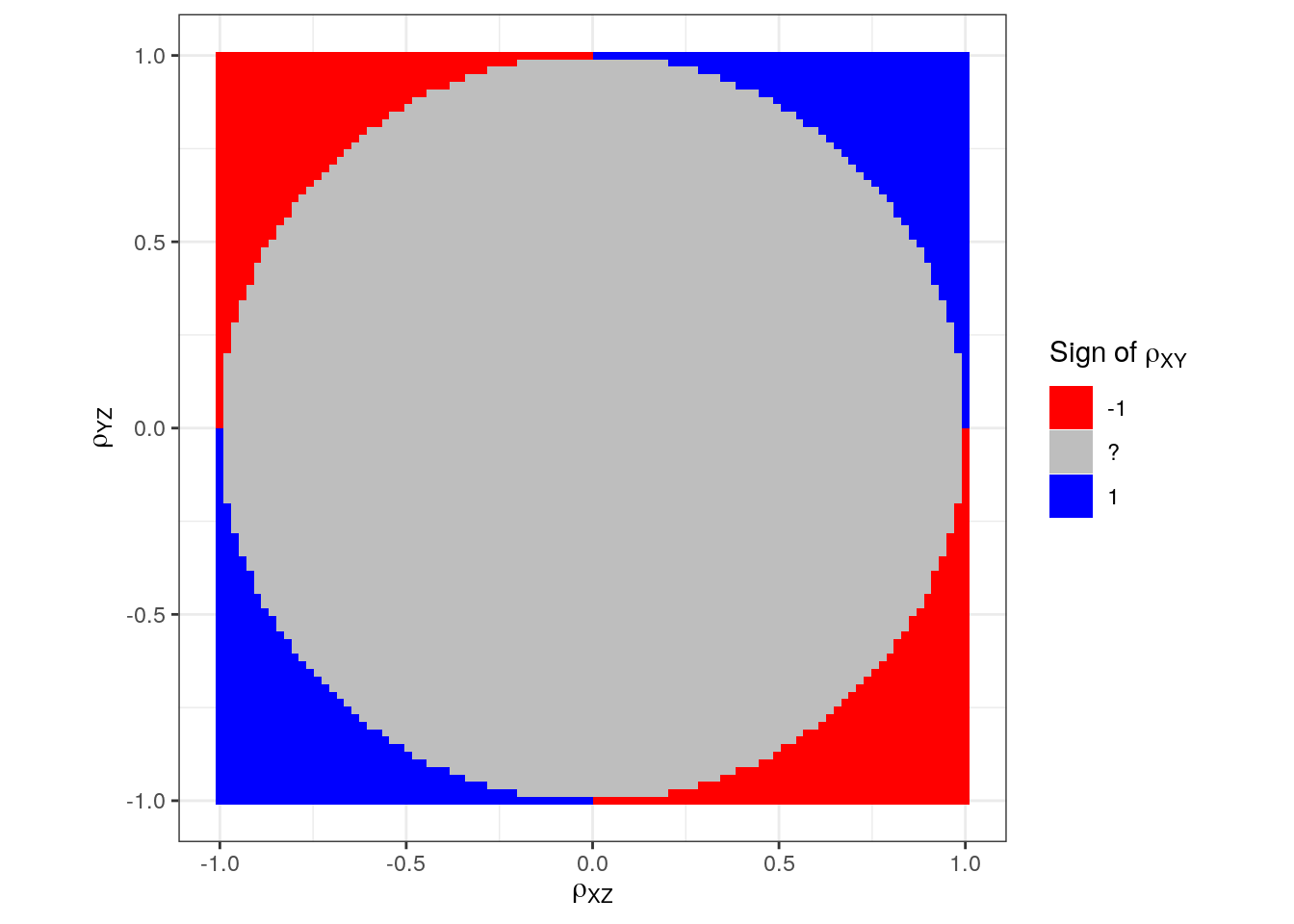Update Aug 10, 2019: I wrote a new blog post about the same as below but using a simulation approach. Update Aug 27, 2019: Minor change in how equations are solved (from version 0.9.0.9122). Let $$\rho_{XY}$$ be the correlation between the stochastic variables $$X$$ and $$Y$$ and similarly for $$\rho_{XZ}$$ and $$\rho_{YZ}$$. If we know two of these, can we say anything...

## How much pizza and how much frozen yogurt?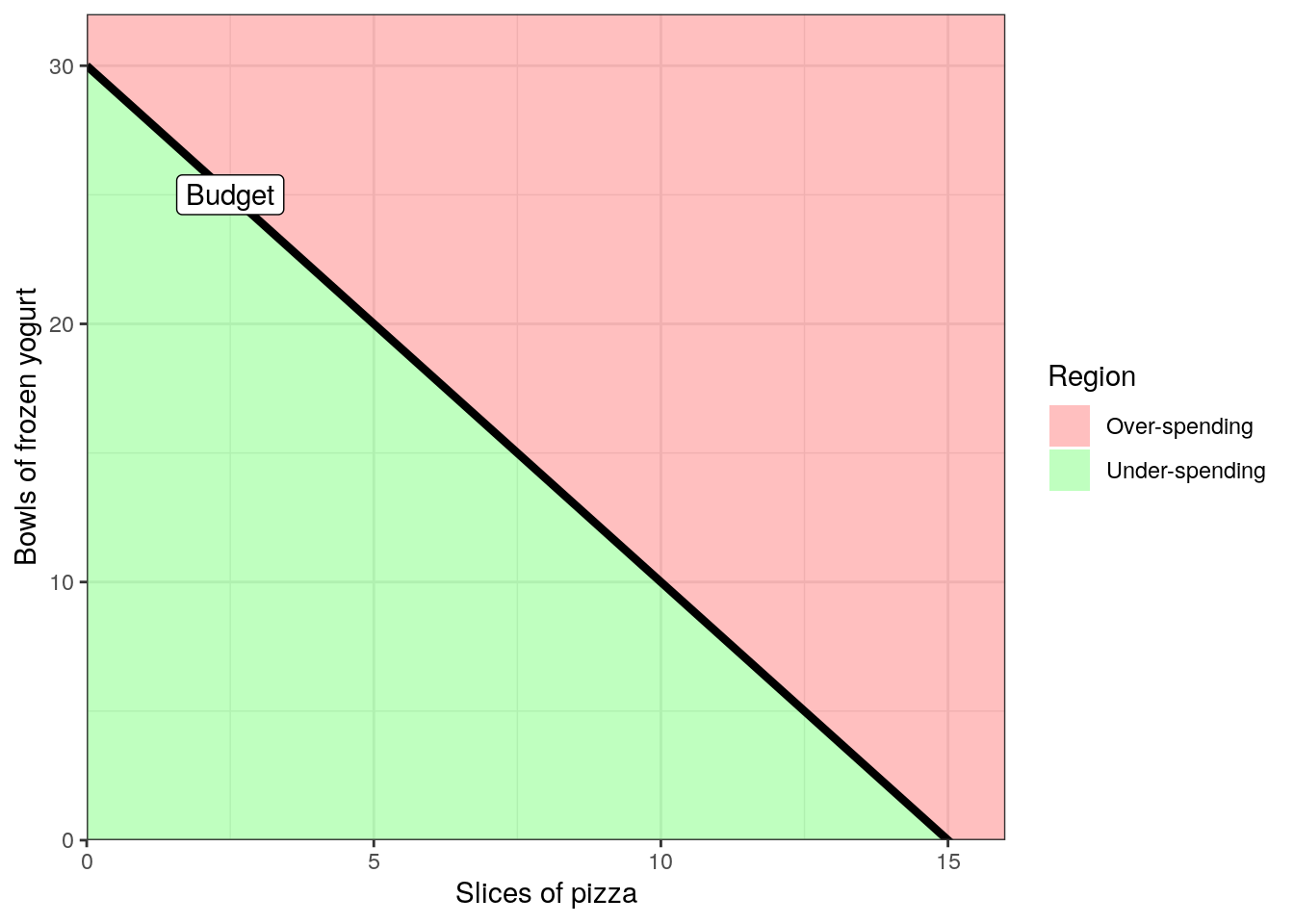Update Aug 27, 2019: I wrote a new blog post showing that Ryacas can now solve the system of equations directly without using optim(). As you may know, I am maintaining the Ryacas package (with online documentation) for doing symbolic mathematics (and other stuff) in R using the yacas software (with online documentation). Søren Højsgaard and I have been preparing a...

## Piano chords in R with the R package ‘pichor’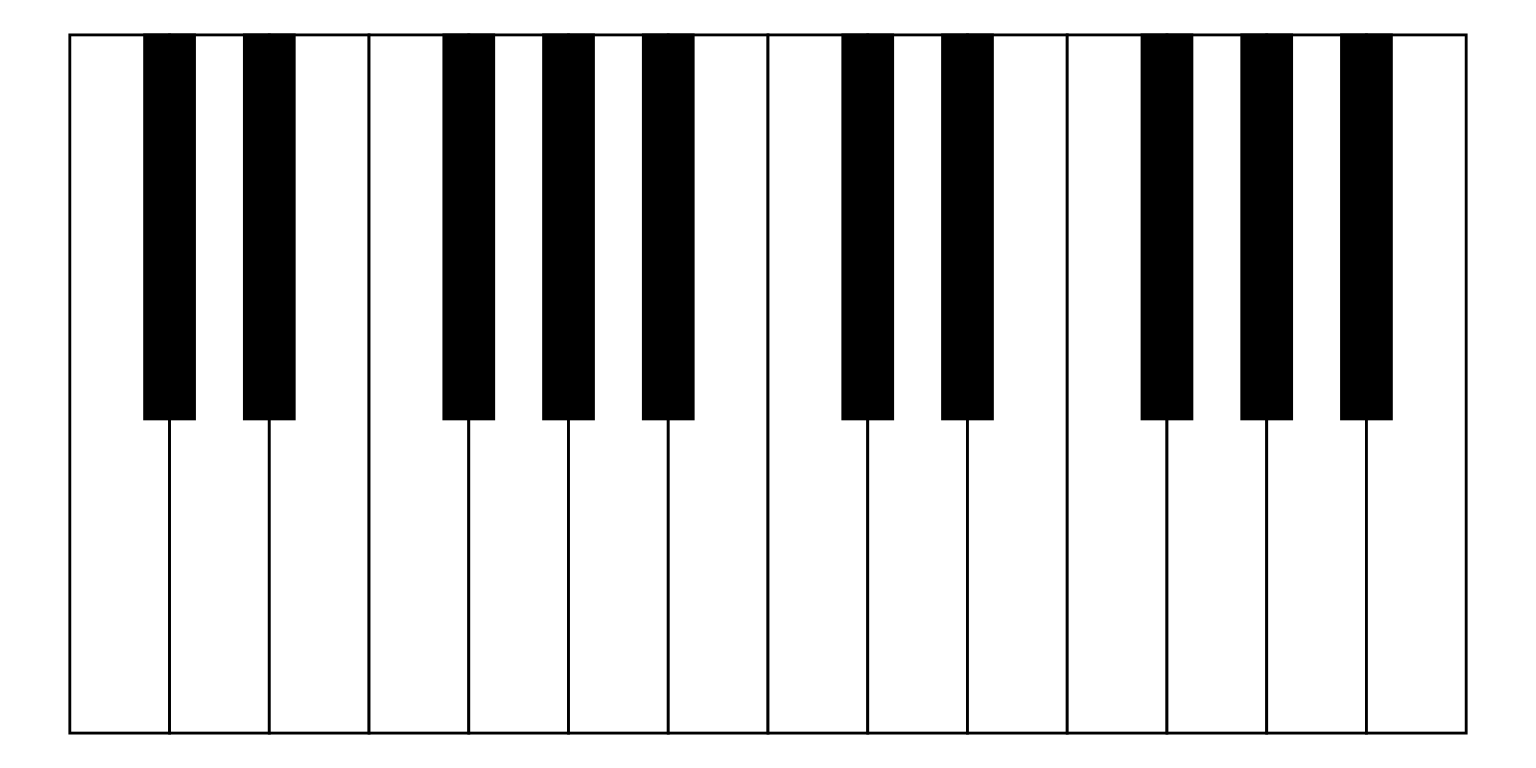I am learning how to play piano. In that process, I needed charts with piano chords. I know it is good practise to construct the chords manually, figure out the inversions and so on, but I found that some aspects of learning were improved with a chord chart. I searched the (entire!) internet, and struggled to find good, customisable charts. Thus I...